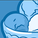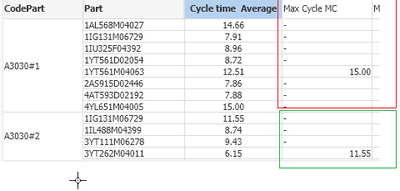Announcements
MAINTENANCE ALERT: Dec. 12th starting 9 AM CET. Community will be read-only. GET DETAILS
cancel
Showing results for
Did you mean:Creator III

## I want all values ​​to be displayed in the table by CodePartI want Max=15 value of CodePart='A3030#1' from every cell in the table.

and Other CodePart also display the Max value. every line in the table

like thisI'm currently trying this code :

Aggr(Max( (Aggr( Sum( Aggr(if(CycleTime_Sec > 0 ,CycleTime_Sec,'0'),Number,WSGroup)/((sum(INPUT) + Sum([INTERNAL])) - sum(Aggr(DISTINCT xWorkOrder, CodePart,Part,Number)) + Sum([TEST])),CodePart,Part))),CodePart)

Labels (7)

• ### Scripting

1 Solution

Accepted SolutionsMVP

Hi

Might be, try like this

Max(Total<CodePart> (Aggr( Sum( Aggr(if(CycleTime_Sec > 0 ,CycleTime_Sec,'0'),Number,WSGroup)/((sum(INPUT) + Sum([INTERNAL])) - sum(Aggr(DISTINCT xWorkOrder, CodePart,Part,Number)) + Sum([TEST])),CodePart,Part)))

Thanks & Regards, Mayil Vahanan R
2 RepliesMVP

Hi

Might be, try like this

Max(Total<CodePart> (Aggr( Sum( Aggr(if(CycleTime_Sec > 0 ,CycleTime_Sec,'0'),Number,WSGroup)/((sum(INPUT) + Sum([INTERNAL])) - sum(Aggr(DISTINCT xWorkOrder, CodePart,Part,Number)) + Sum([TEST])),CodePart,Part)))

Thanks & Regards, Mayil Vahanan R